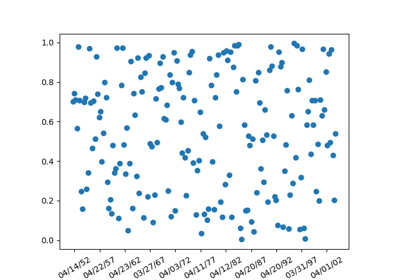You are reading an old version of the documentation (v3.0.0). For the latest version see https://matplotlib.org/stable/api/_as_gen/matplotlib.pyplot.plot_date.html

# matplotlib.pyplot.plot_date¶

`matplotlib.pyplot.``plot_date`(x, y, fmt='o', tz=None, xdate=True, ydate=False, *, data=None, **kwargs)[source]

Plot data that contains dates.

Similar to `plot`, this plots y vs. x as lines or markers. However, the axis labels are formatted as dates depending on xdate and ydate.

Parameters:
x, y : array-like

The coordinates of the data points. If xdate or ydate is True, the respective values x or y are interpreted as Matplotlib dates.

fmt : str, optional

The plot format string. For details, see the corresponding parameter in `plot`.

tz : [ None | timezone string | `tzinfo` instance]

The time zone to use in labeling dates. If None, defaults to rcParam `timezone`.

xdate : bool, optional, default: True

If True, the x-axis will be interpreted as Matplotlib dates.

ydate : bool, optional, default: False

If True, the y-axis will be interpreted as Matplotlib dates.

Returns:
lines

A list of `Line2D` objects representing the plotted data.

Other Parameters:
**kwargs

Keyword arguments control the `Line2D` properties:

Property Description
`agg_filter` a filter function, which takes a (m, n, 3) float array and a dpi value, and returns a (m, n, 3) array
`alpha` float
`animated` bool
`antialiased` bool
`clip_box` `Bbox`
`clip_on` bool
`clip_path` [(`Path`, `Transform`) | `Patch` | None]
`color` color
`contains` callable
`dash_capstyle` {'butt', 'round', 'projecting'}
`dash_joinstyle` {'miter', 'round', 'bevel'}
`dashes` sequence of floats (on/off ink in points) or (None, None)
`drawstyle` {'default', 'steps', 'steps-pre', 'steps-mid', 'steps-post'}
`figure` `Figure`
`fillstyle` {'full', 'left', 'right', 'bottom', 'top', 'none'}
`gid` str
`in_layout` bool
`label` object
`linestyle` {'-', '--', '-.', ':', '', (offset, on-off-seq), ...}
`linewidth` float
`marker` unknown
`markeredgecolor` color
`markeredgewidth` float
`markerfacecolor` color
`markerfacecoloralt` color
`markersize` float
`markevery` unknown
`path_effects` `AbstractPathEffect`
`picker` float or callable[[Artist, Event], Tuple[bool, dict]]
`pickradius` float
`rasterized` bool or None
`sketch_params` (scale: float, length: float, randomness: float)
`snap` bool or None
`solid_capstyle` {'butt', 'round', 'projecting'}
`solid_joinstyle` {'miter', 'round', 'bevel'}
`transform` matplotlib.transforms.Transform
`url` str
`visible` bool
`xdata` 1D array
`ydata` 1D array
`zorder` float

See also

`matplotlib.dates`
Helper functions on dates.
`matplotlib.dates.date2num`
Convert dates to num.
`matplotlib.dates.num2date`
Convert num to dates.
`matplotlib.dates.drange`
Create an equally spaced sequence of dates.

Notes

If you are using custom date tickers and formatters, it may be necessary to set the formatters/locators after the call to `plot_date`. `plot_date` will set the default tick locator to `AutoDateLocator` (if the tick locator is not already set to a `DateLocator` instance) and the default tick formatter to `AutoDateFormatter` (if the tick formatter is not already set to a `DateFormatter` instance).

Note

In addition to the above described arguments, this function can take a data keyword argument. If such a data argument is given, the following arguments are replaced by data[<arg>]:

• All arguments with the following names: 'x', 'y'.

Objects passed as data must support item access (`data[<arg>]`) and membership test (`<arg> in data`).

## Examples using `matplotlib.pyplot.plot_date`¶Date Demo Rrule